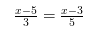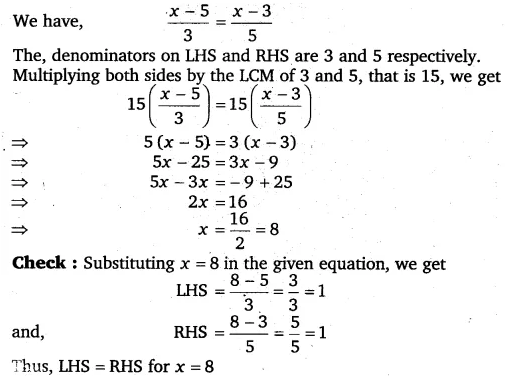# Solve the following linear equations: x-5/3 = x-3/5

Solve the following linear equations: